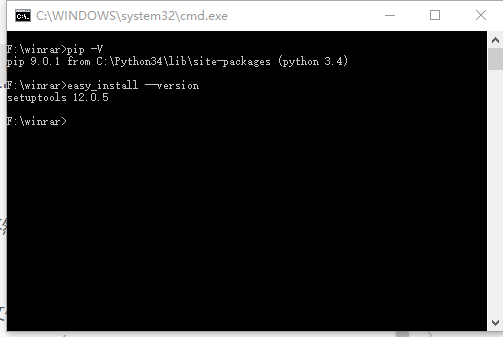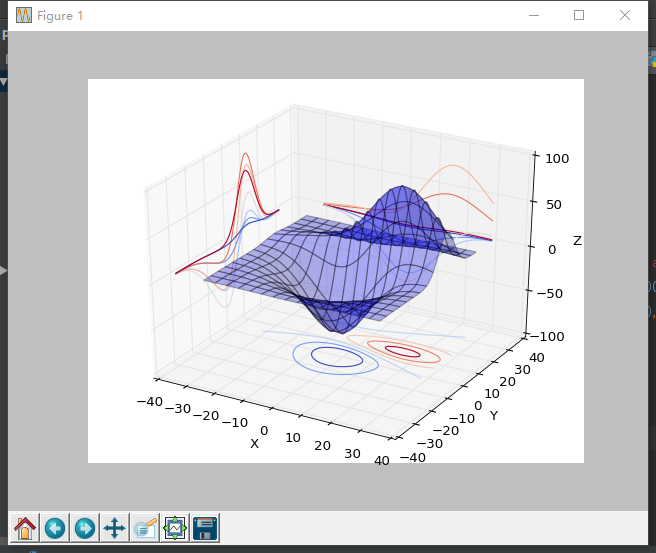# win10下python3.4配置matplotlib

1.下载安装python

2.需要的基本配置

http://blog.csdn.net/u012513525/article/details/54927333

3.安装Matplotlibeasy_install –version (查看easy_install版本)py -m pip install D:\numpy‑1.11.3+mkl‑cp27‑cp27m‑win_amd64.whlpy -m pip install D:\scipy‑0.18.1‑cp27‑cp27m‑win_amd64.whlfrom mpl_toolkits.mplot3d import axes3d
import matplotlib.pyplot as plt
from matplotlib import cm

fig = plt.figure()
ax = fig.gca(projection='3d')
X, Y, Z = axes3d.get_test_data(0.05)
ax.plot_surface(X, Y, Z, rstride=8, cstride=8, alpha=0.3)
cset = ax.contour(X, Y, Z, zdir='z', offset=-100, cmap=cm.coolwarm)
cset = ax.contour(X, Y, Z, zdir='x', offset=-40, cmap=cm.coolwarm)
cset = ax.contour(X, Y, Z, zdir='y', offset=40, cmap=cm.coolwarm)

ax.set_xlabel('X')
ax.set_xlim(-40, 40)
ax.set_ylabel('Y')
ax.set_ylim(-40, 40)
ax.set_zlabel('Z')
ax.set_zlim(-100, 100)

plt.show()ok，现在我们就可以尽情的使用matplotlib了！！!©️2019 CSDN 皮肤主题: 编程工作室 设计师: CSDN官方博客